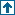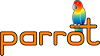parrotcode: Mathematical Operations Contents | Ops

# NAMEmath.ops - Mathematical Operations

# DESCRIPTIONOperations that perform some sort of mathematics, including both basic math and transcendental functions.

###############################################################################

## General infix operationsThese operations take an infix operation number and PMC arguments.

########################################

`infix(inconst INT, in PMC, in INT)`

`infix(inconst INT, in PMC, in NUM)`

`infix(inconst INT, in PMC, in STR)`

`infix(inconst INT, in PMC, in PMC)`

General inplace infix dispatch opcode. \$1 is the MMD function number.

`infix(inconst INT, out PMC, in PMC, in INT)`

`infix(inconst INT, out PMC, in PMC, in NUM)`

`infix(inconst INT, out PMC, in PMC, in STR)`

`infix(inconst INT, out PMC, in PMC, in PMC)`

General infix dispatch opcode. \$1 is the MMD function number.

`n_infix(inconst INT, out PMC, in PMC, in INT)`

`n_infix(inconst INT, out PMC, in PMC, in NUM)`

`n_infix(inconst INT, out PMC, in PMC, in STR)`

`n_infix(inconst INT, out PMC, in PMC, in PMC)`

General infix dispatch opcode. \$1 is the MMD function number. These opcodes return a new result PMC \$2.

## Arithmetic operationsThese operations store the results of arithmetic on other registers and constants into their destination register, \$1.

abs(inout INT)

abs(inout NUM)

abs(in PMC)

Set \$1 to its absolute value.

abs(out INT, in INT)

abs(out NUM, in INT)

abs(out NUM, in NUM)

abs(out PMC, in PMC)

Set \$1 to absolute value of \$2.

n_abs(out PMC, in PMC)

Create new \$1 as the absolute of \$2.

Increase \$1 by the amount in \$2.

add(out INT, in INT, in INT)

add(out NUM, in NUM, in INT)

add(out NUM, in NUM, in NUM)

add(in PMC, in PMC, in INT)

add(in PMC, in PMC, in NUM)

add(in PMC, in PMC, in PMC)

Set \$1 to the sum of \$2 and \$3.

Please note that all PMC variants of all infix functions are handled by the `infix` and `n_infix` opcodes. Thus there is no distinct implementation of e.g. `add_p_p_p`.

cmod(out INT, in INT, in INT)

NOTE: This "uncorrected mod" algorithm uses the C language's built-in mod operator (x % y), which is

```    ... the remainder when x is divided by y, and thus is zero
when y divides x exactly.
...
The direction of truncation for / and the sign of the result
for % are machine-dependent for negative operands, as is the
action taken on overflow or underflow.
-- , page 41```
Also:

```    ... if the second operand is 0, the result is undefined.
Otherwise, it is always true that (a/b)*b + a%b is equal to z. If
both operands are non-negative, then the remainder is non-
negative and smaller than the divisor; if not, it is guaranteed
only that the absolute value of the remainder is smaller than
the absolute value of the divisor.
-- , page 205```
This op is provided for those who need it (such as speed-sensitive applications with heavy use of mod, but using it only with positive arguments), but a more mathematically useful mod based on ** floor(x/y) and defined with y == 0 is provided by the mod op.

```   Brian W. Kernighan and Dennis M. Ritchie, *The C Programming
Language*, Second Edition. Prentice Hall, 1988.```
TODO: Doesn't the Parrot interpreter need to catch the exception?

cmod(in PMC, in PMC, in PMC)

cmod(in PMC, in PMC, in INT)

cmod(in PMC, in PMC, in NUM)

cmod(out NUM, in NUM, in NUM)

NOTE: This "uncorrected mod" algorithm uses the built-in C math library's fmod() function, which computes

```    ... the remainder of dividing x by y. The return value is
x - n * y, where n is the quotient of x / y, rounded towards
zero to an integer.
-- fmod() manpage on RedHat Linux 7.0```

```    the remainder, unless y is zero, when the function fails and
errno is set.```
According to page 251 of , the result when y is zero is implementation- defined.

This op is provided for those who need it, but a more mathematically useful numeric mod based on floor(x/y) instead of truncate(x/y) and defined with y == 0 is provided by the mod op.

```   Brian W. Kernighan and Dennis M. Ritchie, *The C Programming
Language*, Second Edition. Prentice Hall, 1988.```
TODO: Doesn't the Parrot interpreter need to catch the exception?

dec(inout INT)

dec(inout NUM)

dec(in PMC)

Decrease \$1 by one.

div(inout INT, in INT)

div(inout NUM, in INT)

div(inout NUM, in NUM)

div(in PMC, in INT)

div(in PMC, in NUM)

div(in PMC, in PMC)

Divide \$1 by \$2.

div(out INT, in INT, in INT)

div(out NUM, in NUM, in INT)

div(out NUM, in NUM, in NUM)

div(in PMC, in PMC, in INT)

div(in PMC, in PMC, in NUM)

div(in PMC, in PMC, in PMC)

Set \$1 to the quotient of \$2 divided by \$3. In the case of INTVAL division, the result is truncated (NOT rounded or floored).

fdiv(inout INT, in INT)

fdiv(inout NUM, in INT)

fdiv(inout NUM, in NUM)

fdiv(in PMC, in INT)

fdiv(in PMC, in NUM)

fdiv(in PMC, in PMC)

Floor divide \$1 by \$2.

fdiv(out INT, in INT, in INT)

fdiv(out NUM, in NUM, in INT)

fdiv(out NUM, in NUM, in NUM)

fdiv(in PMC, in PMC, in INT)

fdiv(in PMC, in PMC, in NUM)

fdiv(in PMC, in PMC, in PMC)

Set \$1 to the quotient of \$2 divided by \$3. The result is the floor() of the division i.e. the next whole integer towards -inf.

ceil(inout NUM)

Set \$1 to the smallest integral value greater than or equal to \$1.

ceil(out INT, in NUM)

ceil(out NUM, in NUM)

Set \$1 to the smallest integral value greater than or equal to \$2.

floor(inout NUM)

Set \$1 to the largest integral value less than or equal to \$1.

floor(out INT, in NUM)

floor(out NUM, in NUM)

Set \$1 to the largest integral value less than or equal to \$2.

inc(inout INT)

inc(inout NUM)

inc(in PMC)

Increase \$1 by one.

mod(out INT, in INT, in INT)

NOTE: This "corrected mod" algorithm is based on the C code on page 70 of . Assuming correct behavior of the built-in mod operator (%) with positive arguments, this algorithm implements a mathematically convenient version of mod, defined thus:

`  x mod y = x - y * floor(x / y)`
For more information on this definition of mod, see section 3.4 of , pages 81-85.

References:

```   Donald E. Knuth, *MMIXware: A RISC Computer for the Third
Millennium* Springer, 1999.

 Ronald L. Graham, Donald E. Knuth and Oren Patashnik, *Concrete
mod(in PMC, in PMC, in PMC)

mod(in PMC, in PMC, in INT)

mod(in PMC, in PMC, in NUM)

Sets \$1 to the modulus of \$2 and \$3.

mod(in PMC, in INT)

Sets \$1 to the modulus of \$1 and \$2.

mod(out NUM, in NUM, in NUM)

NOTE: This "corrected mod" algorithm is based on the formula of :

`  x mod y = x - y * floor(x / y)`
For more information on this definition of mod, see section 3.4 of , pages 81-85.

References:

```   Ronald L. Graham, Donald E. Knuth and Oren Patashnik, *Concrete
mul(inout INT, in INT)

mul(inout NUM, in INT)

mul(inout NUM, in NUM)

mul(in PMC, in INT)

mul(in PMC, in NUM)

mul(in PMC, in PMC)

Set \$1 to the product of \$1 and \$2.

mul(out INT, in INT, in INT)

mul(out NUM, in NUM, in INT)

mul(out NUM, in NUM, in NUM)

mul(in PMC, in PMC, in INT)

mul(in PMC, in PMC, in NUM)

mul(in PMC, in PMC, in PMC)

Set \$1 to the product of \$2 and \$3.

neg(inout INT)

neg(inout NUM)

neg(in PMC)

Set \$1 to its negative.

neg(out INT, in INT)

neg(out NUM, in NUM)

neg(out PMC, in PMC)

Set \$1 to the negative of \$2.

n_neg(out PMC, in PMC)

Create \$1 as the negative of \$2.

pow(out NUM, in INT, in INT)

pow(out NUM, in INT, in NUM)

pow(out NUM, in NUM, in INT)

pow(out NUM, in NUM, in NUM)

pow(in PMC, in PMC, in PMC)

pow(in PMC, in PMC, in NUM)

pow(in PMC, in PMC, in INT)

Set \$1 to \$2 raised to the power \$3.

sub(inout INT, in INT)

sub(inout NUM, in INT)

sub(inout NUM, in NUM)

sub(in PMC, in INT)

sub(in PMC, in NUM)

sub(in PMC, in PMC)

Decrease \$1 by the amount in \$2.

sub(out INT, in INT, in INT)

sub(out NUM, in NUM, in INT)

sub(out NUM, in NUM, in NUM)

sub(in PMC, in PMC, in INT)

sub(in PMC, in PMC, in NUM)

sub(in PMC, in PMC, in PMC)

Set \$1 to \$2 minus \$3.

sqrt(out NUM, in INT)

sqrt(out NUM, in NUM)

Set \$1 to the square root of \$2.

Please note that for all these opcodes the variant with an INTVAL argument is deprecated. Actually code is generated that uses a FLOATVAL argument instead.

## Transcendental mathematical operationsThese operations perform various transcendental operations such as logarithmics and trigonometrics.

acos(out NUM, in INT)

acos(out NUM, in NUM)

Set \$1 to the arc cosine (in radians) of \$2.

asec(out NUM, in INT)

asec(out NUM, in NUM)

Set \$1 to the arc secant (in radians) of \$2.

asin(out NUM, in INT)

asin(out NUM, in NUM)

Set \$1 to the arc sine (in radians) of \$2.

atan(out NUM, in INT)

atan(out NUM, in NUM)

atan(out NUM, in INT, in INT)

atan(out NUM, in INT, in NUM)

atan(out NUM, in NUM, in INT)

atan(out NUM, in NUM, in NUM)

The two-argument versions set \$1 to the arc tangent (in radians) of \$2.

The three-argument versions set \$1 to the arc tangent (in radians) of \$2 / \$3, taking account of the signs of the arguments in determining the quadrant of the result.

cos(out NUM, in INT)

cos(out NUM, in NUM)

Set \$1 to the cosine of \$2 (given in radians).

cosh(out NUM, in INT)

cosh(out NUM, in NUM)

Set \$1 to the hyperbolic cosine of \$2 (given in radians).

exp(out NUM, in INT)

exp(out NUM, in NUM)

Set \$1 to e raised to the power \$2. e is the base of the natural logarithm.

ln(out NUM, in INT)

ln(out NUM, in NUM)

Set \$1 to the natural (base e) logarithm of \$2.

log10(out NUM, in INT)

log10(out NUM, in NUM)

Set \$1 to the base 10 logarithm of \$2.

log2(out NUM, in INT)

log2(out NUM, in NUM)

Set \$1 to the base 2 logarithm of \$2.

sec(out NUM, in INT)

sec(out NUM, in NUM)

Set \$1 to the secant of \$2 (given in radians).

sech(out NUM, in INT)

sech(out NUM, in NUM)

Set \$1 to the hyperbolic secant of \$2 (given in radians).

sin(out NUM, in INT)

sin(out NUM, in NUM)

Set \$1 to the sine of \$2 (given in radians).

sinh(out NUM, in INT)

sinh(out NUM, in NUM)

Set \$1 to the hyperbolic sine of \$2 (given in radians).

tan(out NUM, in INT)

tan(out NUM, in NUM)

Set \$1 to the tangent of \$2 (given in radians).

tanh(out NUM, in INT)

tanh(out NUM, in NUM)

Set \$1 to the hyperbolic tangent of \$2 (given in radians).

## Other mathematical operationsImplementations of various mathematical operations

gcd(out INT, in INT, in INT)

Greatest Common divisor of \$2 and \$3.

lcm(out INT, in INT, in INT)

Least Common Multiple of \$2 and \$3

fact(out INT, in INT)

fact(out NUM, in INT)

Factorial, n!. Calculates the product of 1 to N.

# LICENSEThis program is free software. It is subject to the same license as the Parrot interpreter itself.

# POD ERRORSHey! The above document had some coding errors, which are explained below:

Around line 27:

You can't have =items (as at line 33) unless the first thing after the =over is an =item